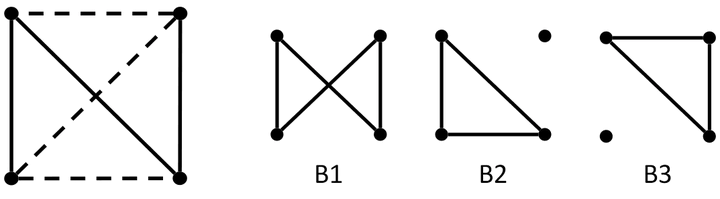# On a Wider Class of Prior Distributions for Graphical ModelsA fundamental system of graph cycles.

### Abstract

Gaussian graphical models are useful tools for conditional independence structure inference of multivariate random variables. Unfortunately, Bayesian inference of latent graph structures is challenging due to exponential growth of $\mathcal{G}_n$, the set of all graphs in n vertices. One approach that has been proposed to tackle this problem is to limit search to subsets of $\mathcal{G}_n$. In this paper, we study subsets that are vector subspaces with the cycle space $C_n$ as main example. We propose a novel prior on $C_n$ based on linear combinations of cycle basis elements and present its theoretical properties. Using this prior, we implemented a Markov chain Monte Carlo algorithm and show that (i) posterior edge inclusion estimates compared to the standard technique are comparable despite searching a smaller graph space and (ii) the vector space perspective enables straightforward MCMC algorithms.

Publication
Journal of Applied Probability##### Abhinav Natarajan
###### Doctoral student in mathematics

My research interests are in applied algebraic topology and geometry, statistics, and machine learning.